# 1. 神经网络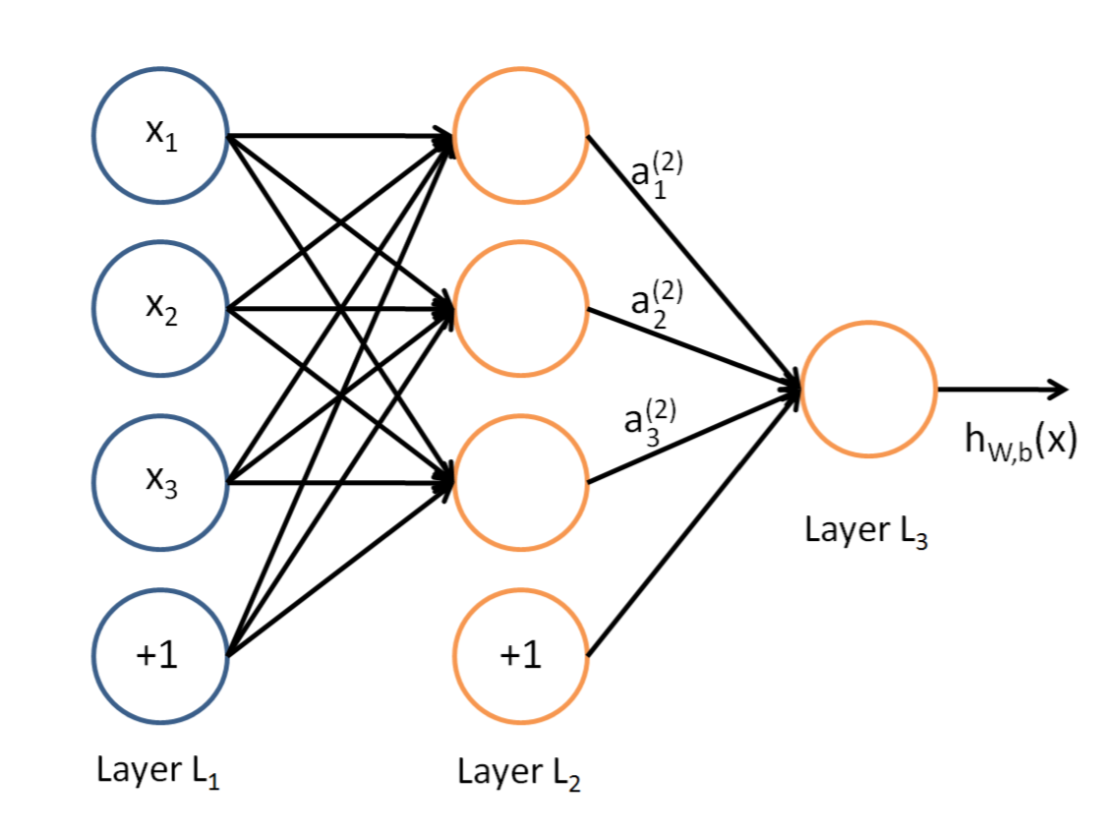# 2.  如何计算传播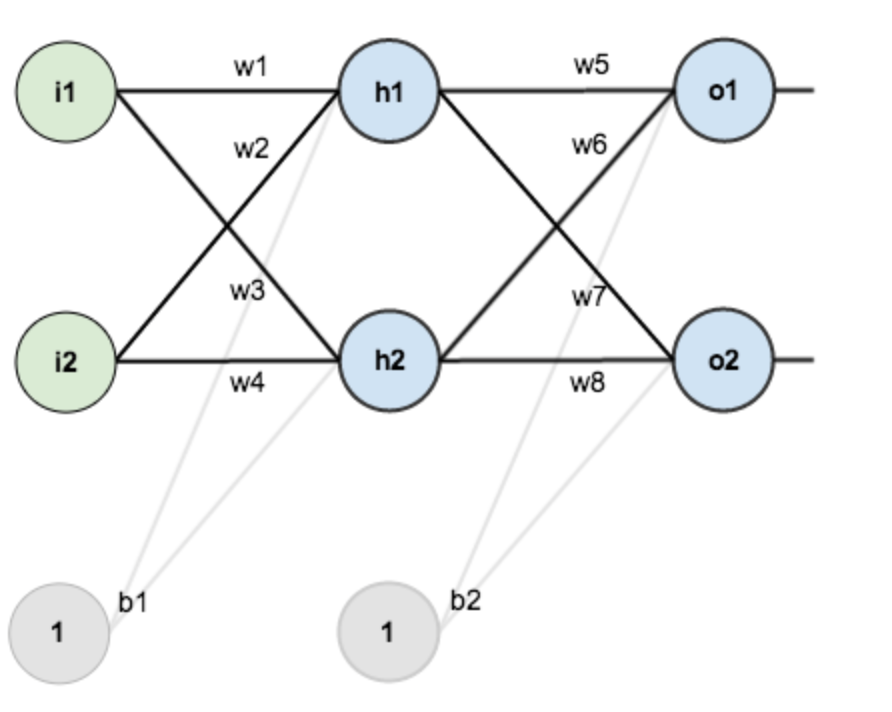• 第一层输入层：里面包含神经元i1,i2，截距：b1，权重：w1,w2,w3,w4
• 第二层是隐含层：里面包含h1,h2，截距：b2，权重：w5,w6,w7,w8
• 第三层是输出层：里面包含o1,o2

w1=0.25
w2=0.25
w3=0.15
w4=0.20
w5=0.30
w6=0.35
w7=0.40
w8=0.35

## 2.1 前向传播

### 2.1.1 输入层到隐含层

NET(h1)=w1*i1+w2*i2+b1=0.25*0.02+0.25*0.04+0.4=0.005+0.01+0.4=0.415

OUT(h1)=1/(1+e^(-NET(h1)))=1/(1+0.660340281)=0.602286177

NET(h2)=w3*i1+w4*i2+b1=0.15*0.02+0.20*0.04+0.4=0.003+0.008+0.4=0.411
OUT(h2)=1/(1+e^(-NET(h2)))=1/(1+0.662986932)=0.601327636

### 2.1.2 从隐含层到输出层

NET(o1)=w5*h1+w6*h2+b2=0.3*0.602286177+0.35*0.601327636+0.7=0.180685853+0.210464672+0.7=1.091150525
OUT(o1)=1/(1+e^(-NET(o1)))=1/(1+0.335829891)=0.748598311

NET(o2)=w7*h1+w8*h2+b2=0.4*0.602286177+0.35*0.601327636+0.7=0.240914471+0.210464672+0.7=1.151379143
OUT(o2)=1/(1+e^(-NET(o2)))=1/1.316200383=0.759762733

o1:0.748598311   o2:0.759762733 距离我们期望的o1:0.5  o2:0.9还是有很大的距离

## 2.2 计算总误差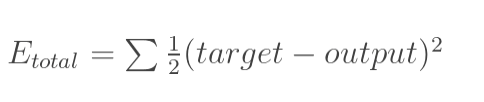E(total)= E(o0)+E(o1)=(1/2)*(0.748598311-0.5)^2+(1/2)*(0.759762733-0.9)^2=0.01545028+0.009833246=0.025283526

## 2.3 反向传播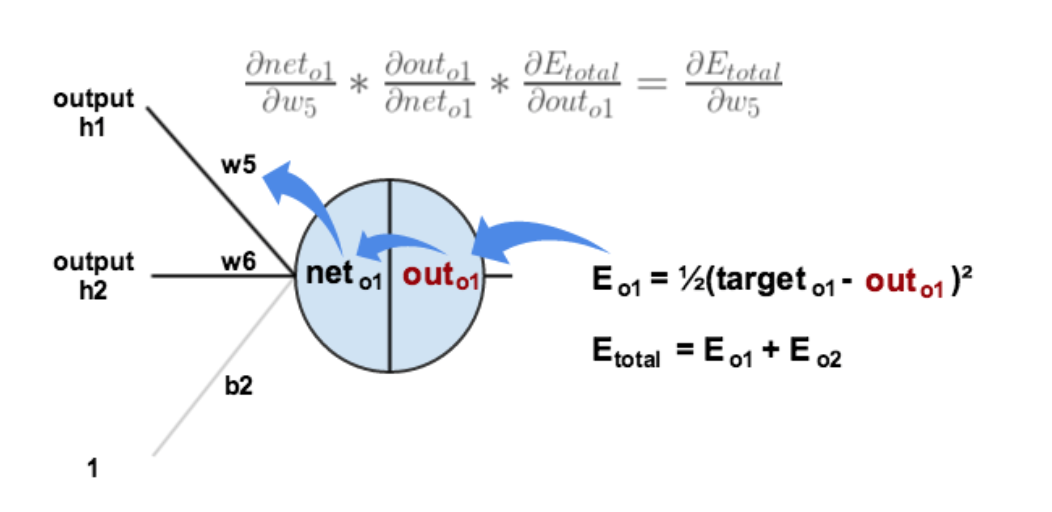### 2.3.1 隐含层到输出层的权值更新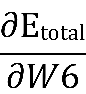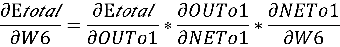• 计算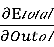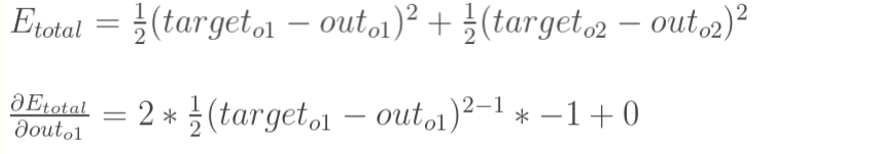为两个关于可导函数，则复合函数的导数为：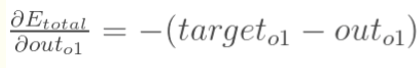=-(0.5-0.748598311)=0.248598311

• 计算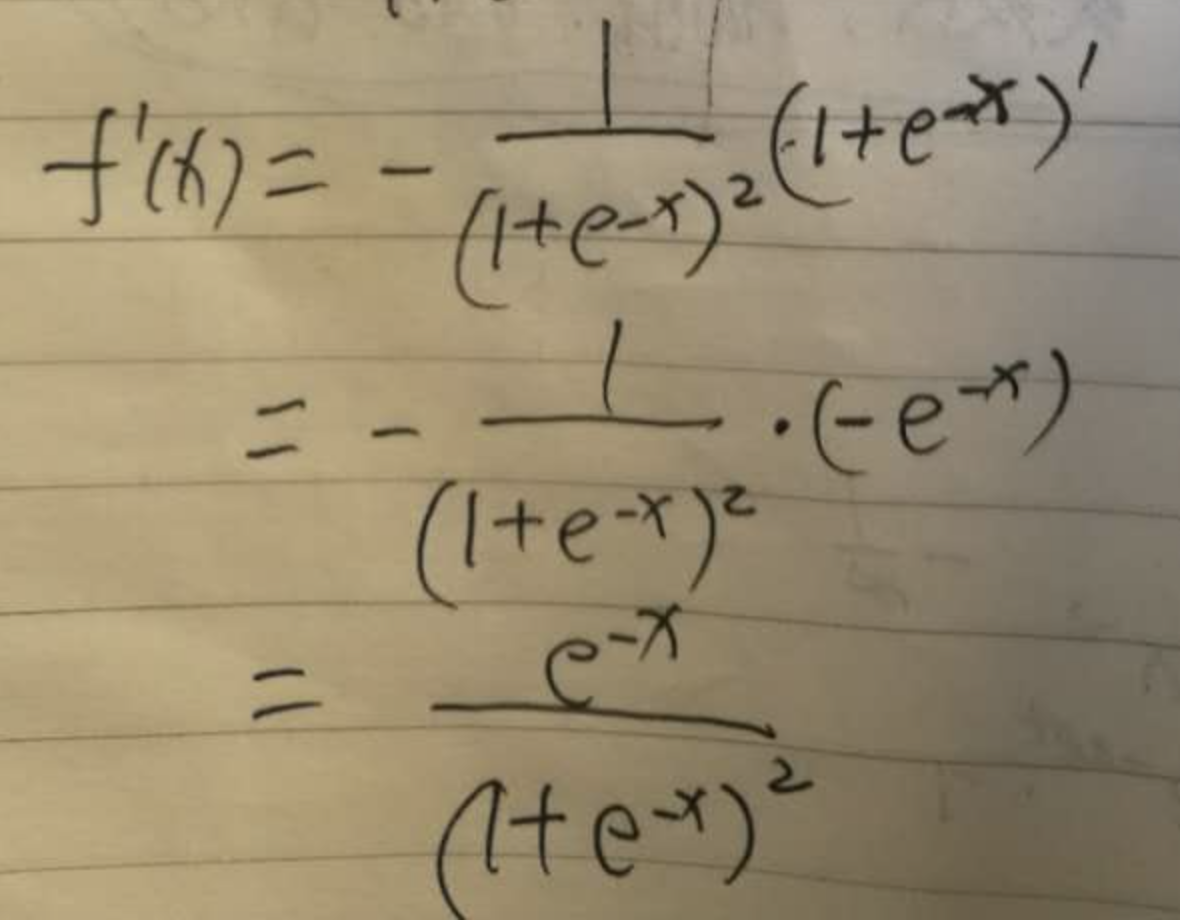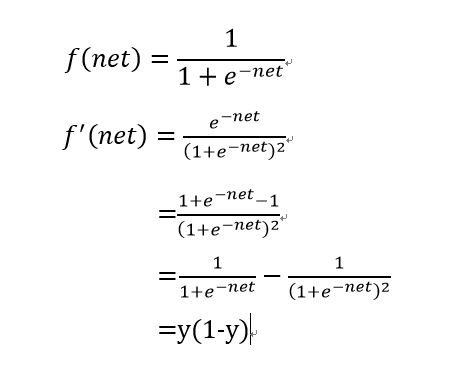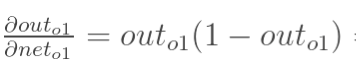=0.748598311*(1-0.748598311)=0.251401689*0.748598311=0.18819888

• 计算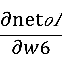=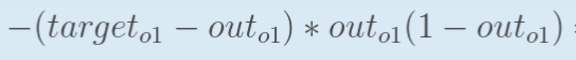*out(h2)

=0.248598311*0.18819888*0.601327636=0.028133669

#### 2.3.1.1 跟新W6的权重

W6=W6-x*0.35-0.1*0.028133669=0.347186633

### 2.3.2 隐含层的权值跟新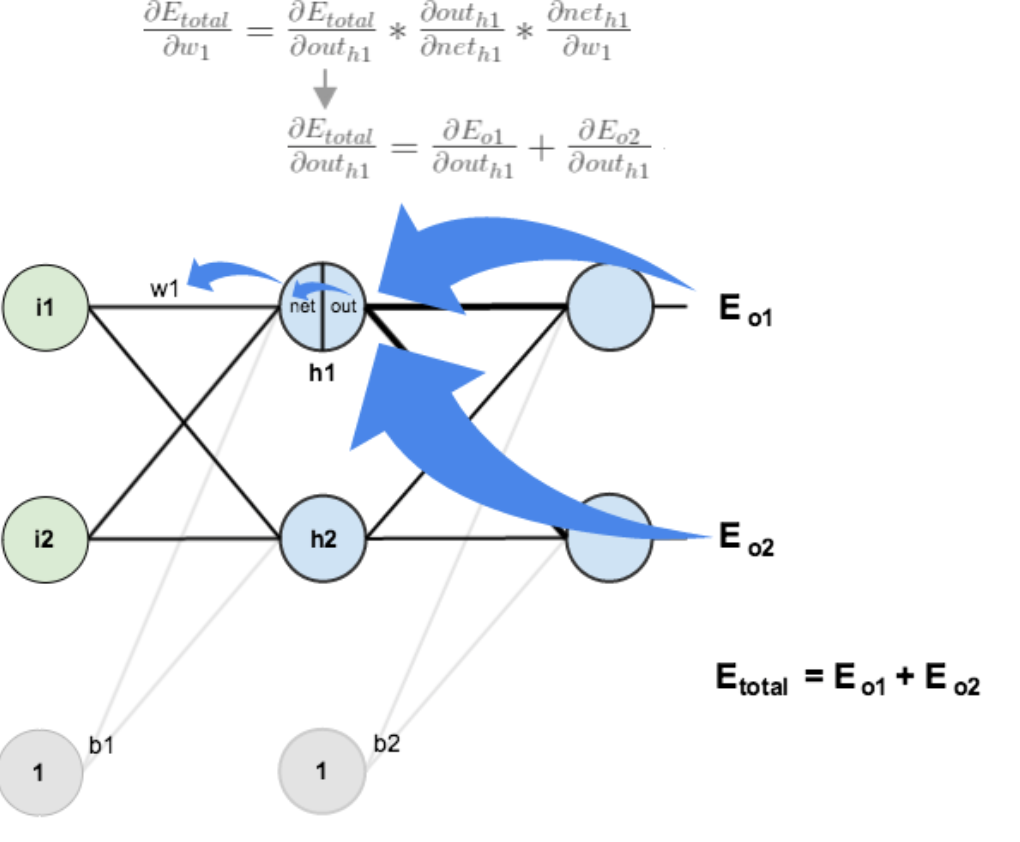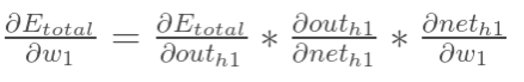#### 2.3.2.1 计算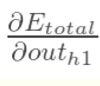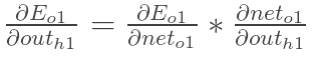• 计算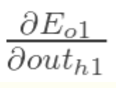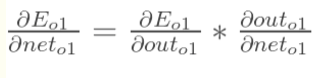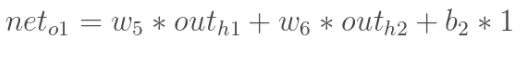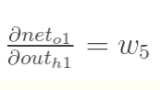• 同理也可以计算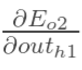#### 2.3.2.2 计算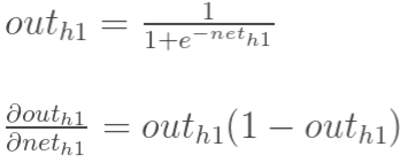#### 2.3.2.3 计算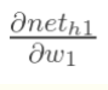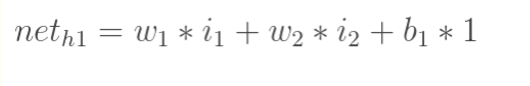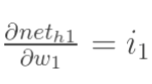#### 2.3.2.4 整体公式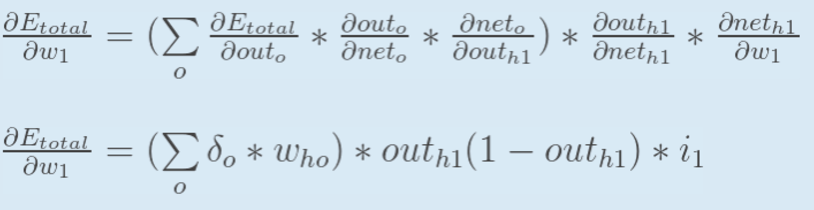#### 2.3.2.4 跟新W1的权重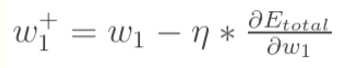# 3. 计算获取最佳的权重